# GMAT Math : DSQ: Calculating the length of the hypotenuse of a right triangle : Pythagorean Theorem

## Example Questions

### Example Question #1 : Dsq: Calculating The Length Of The Hypotenuse Of A Right Triangle : Pythagorean Theorem

Two ships left New York at the same time. One ship has been moving due east the entire time at a speed of 50 nautical miles per hour. How far apart are the ships now?

1) The other ship has been moving due south the entire time.

2) The other ship has been moving at a rate of 60 nautical miles per hour the entire time.

Statement 2 ALONE is sufficient to answer the question, but Statement 1 ALONE is not sufficient to answer the question.
EITHER statement ALONE is sufficient to answer the question.
BOTH statements TOGETHER are not sufficient to answer the question.
Statement 1 ALONE is sufficient to answer the question, but Statement 2 ALONE is not sufficient to answer the question.
BOTH statements TOGETHER are sufficient to answer the question, but NEITHER statement ALONE is sufficient to answer the question.
Correct answer: BOTH statements TOGETHER are not sufficient to answer the question.
Explanation:

In order to determine how far apart the ships are now, it is necessary to know how far each ship is from New York now. Even knowing both statements, however, you only know that the paths are at right angles, and that the ratio of the two distances is 6 to 5. Without knowing the time elapsed, which is not given, you cannot tell how far apart the ships are.

The answer is that both statements together are insufficent to answer the question.

### Example Question #2 : Dsq: Calculating The Length Of The Hypotenuse Of A Right Triangle : Pythagorean Theorem

Right triangle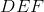is similar to triangle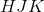.

Find the hypotenuse of triangleI) Trianglehas side lengths of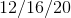.

II) The shortest side ofis.

Statement 1 is sufficient to solve the question, but statement 2 is not sufficient to solve the question.

Statement 2 is sufficient to solve the question, but statement 1 is not sufficient to solve the question.

Neither statement is sufficient to solve the question. More information is needed.

Both statements taken together are sufficient to solve the question.

Each statement alone is enough to solve the question.

Both statements taken together are sufficient to solve the question.

Explanation:

We need both statements here because we need to work with ratios.

If you notice that a 12/16/20 triangle follows the 3/4/5 triangle ratio, then it is even easier. Otherwise, you can set up the following proportion and solve to find the answer.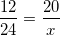Where x is the hypotenuse of triangle HJK.

### Example Question #3 : Dsq: Calculating The Length Of The Hypotenuse Of A Right Triangle : Pythagorean Theorem

Find the hypotenuse of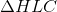.

I) The shortest side is half of the second shortest side.

II) The middle side isfathoms long.

Statement II is sufficient to answer the question, but statement I is not sufficient to answer the question.

Either statement is sufficient to answer the question.

Statement I is sufficient to answer the question, but statement II is not sufficient to answer the question.

Both statements are needed to answer the question.

Both statements are needed to answer the question.

Explanation:

To find the hypotenuse of a right triangle we can use the classic Pythagorean Theorem. To do so, however, we need to know the other two sides.

I) Tells us how to relate the other two sides to eachother.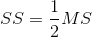II) Gives us the length of one side.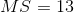Use II) and I) to find the two short sides. Then use Pythagorean Theorem to find the perimeter.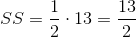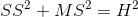, where SS represents the shortest side, MS represents the middle side, and H represents the hypotenuse.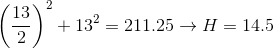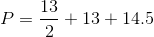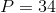### Example Question #4 : Dsq: Calculating The Length Of The Hypotenuse Of A Right Triangle : Pythagorean Theorem

Calculate the length of the hypotenuse of a right triangle.

1. The area of the right triangle is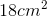.
2. One of the angles is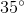.

Statement 1 alone is sufficient, but statement 2 alone is not sufficient to answer the question.

Both statements taken together are sufficient to answer the question, but neither statement alone is sufficient.

Statements 1 and 2 are not sufficient, and additional data is needed to answer the question.

Each statement alone is sufficient to answer the question.

Statement 2 alone is sufficient, but statement 1 alone is not sufficient to answer the question.

Statements 1 and 2 are not sufficient, and additional data is needed to answer the question.

Explanation:

Statement 1:In order to find the hypotenuse, we need to find the values for the base and length. Although we're given the area, we need additional information.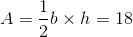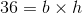but the base and height can be 2 and 183 and 124 and 9, or 6 and 6.

Statement 2: We don't need this information to find the length of the hypotenuse.

Statements 1 and 2 are not sufficient, and additional data is needed to answer the question.

### Example Question #5 : Dsq: Calculating The Length Of The Hypotenuse Of A Right Triangle : Pythagorean Theorem

Calculate the length of the hypotenuse of the right triangle.

1. The area of the right triangle is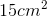.
2. The difference between the height and base is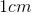.

Both statements taken together are sufficient to answer the question, but neither statement alone is sufficient.

Statement 1 alone is sufficient, but statement 2 alone is not sufficient to answer the question.

Each statement alone is sufficient to answer the question.

Statement 2 alone is sufficient, but statement 1 alone is not sufficient to answer the question.

Statements 1 and 2 are not sufficient, and additional data is needed to answer the question.

Both statements taken together are sufficient to answer the question, but neither statement alone is sufficient.

Explanation:

Statement 1: We're given the area but require additional information.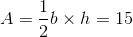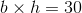The base and height, however, can be 2 and 153 and 10, or 5 and 6.

Statement 2: We're provided the information that narrows our possible values. The difference between the base and height isso our values must be 5 and 6

Using BOTH statements, we can find the hypotenuse of the right triangle via the Pythagorean Theorem: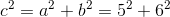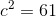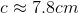Both statements taken together are sufficient to answer the question, but neither statement alone is sufficient.

### Example Question #6 : Dsq: Calculating The Length Of The Hypotenuse Of A Right Triangle : Pythagorean Theorem

Find the length of the hypotenuse of the right triangle.

1. The area of the triangle is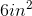.
2. The legs are measured to be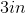and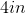.

Statement 1 alone is sufficient, but statement 2 alone is not sufficient to answer the question.

Both statements taken together are sufficient to answer the question, but neither statement alone is sufficient.

Statements 1 and 2 are not sufficient, and additional data is needed to answer the question.

Statement 2 alone is sufficient, but statement 1 alone is not sufficient to answer the question.

Each statement alone is sufficient to answer the question.

Statement 2 alone is sufficient, but statement 1 alone is not sufficient to answer the question.

Explanation: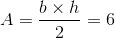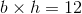Which means the base and height can either be and 3, or 6 and 2. We cannot find the length of the hypotenuse unless we know which one.

Statement 2: We're given the lengths of the base and height so we can just plug them into the Pythagorean Theorem.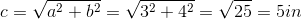Tired of practice problems?

Try live online GMAT prep today.